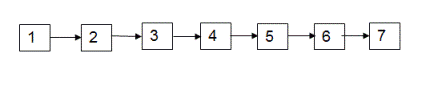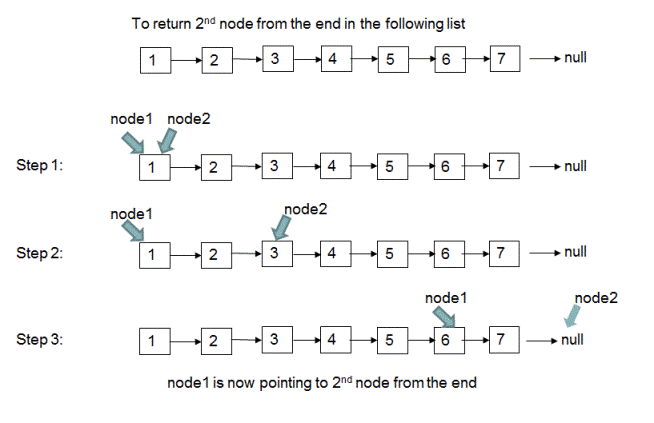# Find nth Node from the end of a Linked List

Given a linked list, find 'n'th node from the end for a given value of n (n > 0). For example, for the following linked listif n = 2, we have to find 2nd node from the end which is node 6.
if n = 1, we have to find 1st node from the end which is node 7.

## Algorithm/Insights

Method 1: For finding out 'n'th node from the end, find the length of given linked list(say lengthOfList) and return (lengthOfList - n + 1)th node from the beginning. For example in the following list of length 7,to return 2nd node from the end(n = 2), we need to return 6th (7 - 2 + 1) node from beginning which is node '6'. This would require two traversals of a given linked list. First traversal to find out the length of the list and second traversal to find the required node.

Method 2: In this method, we use only one traversal of the given linked list to find out 'n'th node from the end. Here we use two references to nodes - 'node1' and 'node2'.
1. We make 'node1' and 'node2' point to first node of the list.
2. We advance 'node1' by 'n' nodes(to find out 'n'th node from last). Basically 'node1' would be pointing to (n+1)th node from the beginning.
3. If 'node1' is not pointing to (n+1)th node and has already reached the end of the list then we know that number of nodes in the given list is less than 'n' and hence 'n'th node from the end cannot be found in this case. We simply return -1 to indicate that.
4. Now knowing that 'node1' is pointing to (n+1)th node and 'node2' is pointing to the first node in the list, we advance both references('node1' and 'node2') simultaneously one node at a time until 'node1' reaches the end of the list. When 'node1' reaches the end of the list, 'node2' would be pointing to the node which is 'n'th from the end. This is because when we started advancing references 'node1' and 'node2', difference between them was of 'n' nodes which is maintained while advancing these references.
5. Because 'node2' is pointing to 'n'th node from the end, we simply return value of node which 'node2' points to.

Example:Please checkout function 'findNthFromEnd(int n)' for implementation details. Time complexity of this algorithm is O(n) and extra space used is O(1).

## Code Snippet

```
package com.ideserve.nilesh.questions;

/**
* <b>IDeserve <br>
* Finds 'n'th node from the end of a given linked list.
* @author Nilesh
*/

public class NthNodeFromLast
{
private class ListNode
{
int value;
ListNode next;

ListNode(int value)
{
this.value = value;
}
}

ListNode tail;

public NthNodeFromLast()
{

}

// appends node at the end of the list
private void appendNode(int value)
{
{
return;
}

ListNode n = new ListNode(value);
tail.next = n;
tail = n;
}

{
for (int i = 1; i <= 7; i++)
{
appendNode(i);
}
}

public void printList()
{

while (temp != null)
{
System.out.print(temp.value + "->");
temp = temp.next;
}
System.out.print("null");
}

public int findNthFromEnd(int n)
{

int count = 1;

// make 'node1' point to (n+1)th node from the beginning
while (node1 != null)
{
if (count == (n+1))
{
break;
}

count += 1;
node1 = node1.next;
}

// if 'node1' is pointing to (n+1)th node from the beginning
// advance both 'node1' and 'node2' - one node at a time
// when node1 reaches at the end of the list, node2 would be pointing to 'n'th node from the last
if (count == (n+1))
{
while (node1 != null)
{
node1 = node1.next;
node2 = node2.next;
}

return node2.value;
}

// if the length of the list is less than 'n'
else
{
System.out.println("\nError: The length of the list is less than " + n);
return -1;
}
}

public static void main(String[] args)
{
NthNodeFromLast solution = new NthNodeFromLast();

solution.printList();

System.out.println("\n\nrequired 'n'th node from the last is: " + solution.findNthFromEnd(2));
}
}
```

## Order of the Algorithm

Time Complexity is O(n)
Space Complexity is O(1)